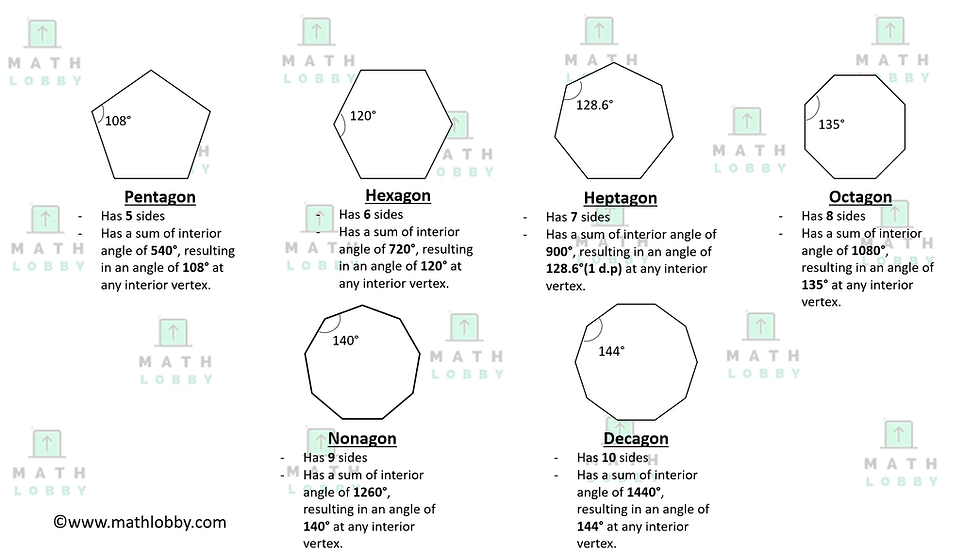top of page
Search

# Polygons in Geometry

Updated: Jun 22, 2021

Dear students of Math Lobby, we will be covering the topic on Polygons in Geometry today. Do check out our other articles Geometry articles namely Angles in Geometry, Triangles in Geometry and Special Quadrilaterals in Geometry if you have not done so. Without further ado, let’s begin!

In this note, you will learn:

1. What are polygons?

2. Types of polygons and its properties

3. Angles involving polygons (Exterior and interior)

### 1. What are polygons?

Polygons are defined to be plane figures with at least three or more straight sides, but typically five and above. It can be further categorized into regular polygons and irregular polygons, but we will be focusing on just regular polygons today. Circles, any shapes that includes a curve, any shape that is not closed and three-dimensional objects cannot be counted as polygons.### Types of polygons and its properties

As mentioned above, we will only be touching regular polygons today as stated in the SEAB syllabus. The types of polygons we will be going through are: Pentagon (5-sided), Hexagon (6-sided), Heptagon (7-sided), Octagon (8-sided), Nonagon (9-sided) and Decagon (10-sided).As you can see from the diagram above, these polygons are called regular polygons, which means that they each have 1) sides of equal length and 2) identical angles at every vertex within the polygon. The general rule of thumb for the sum of interior angles in a polygon and the interior angle at each vertex of a polygon is given by:

Sum of interior angles = (n - 2) x 180°

Interior angle at each vertex of a polygon = [(n – 2) x 180°] / n

where n = number of sides of the polygon

Question Time!

Can you find the sum of interior angles and interior angle at each vertex of a 52-sided polygon? Leave your answer in the comments down below!Exterior angle of a polygon is defined to be the angle between any side of a polygon, and the line extended from the next side. Since we know that angles on a straight line must be equals to 180°, to know the value of the exterior angle, we just have to simply deduct the interior angle with 180°.

However, there is another rule: The sum of all exterior angles of a polygon will always add up to 360°. Hence, this also means:

Exterior angle at each vertex of a polygon = 360° / n,

where n = number of sides of the polygon

Now, let’s put on our thinking cap and get our problem-solving skills moving!

1. How many sides would a regular polygon need to have an exterior angle of 8°?2. Given the diagram above, find the value of both x and y. (Corrected to 1 decimal place wherever applicable)3. The “Math Lobby” logo has an arrow in the center, and it can be categorized as an irregular polygon. It can be reflected about line A. With the information provided in the diagram, find the value of x.

*Hint: Although the arrow is an irregular polygon, the only special characteristic it has is that it’s interior and exterior angles are not all equal, but the sum of the interior angles is still respective to the number of sides it has!

And that’s all for today, students! Math Lobby hopes that after this article, you have a better understanding of the different types and properties of polygons, and a clear idea on when to apply the formulae! If you have any pending questions, please do go on to our Facebook page, Instagram or contact us directly at Math Lobby! We have certified mathematics tutors to aid you in your journey to becoming a better student!

As always: Work hard, stay motivated and I wish all students a successful and enjoyable journey with Math Lobby!

If you want to receive daily Secondary Math Tips from us,

Visit and FOLLOW our Instagram page at https://www.instagram.com/mathlobbymotivation/